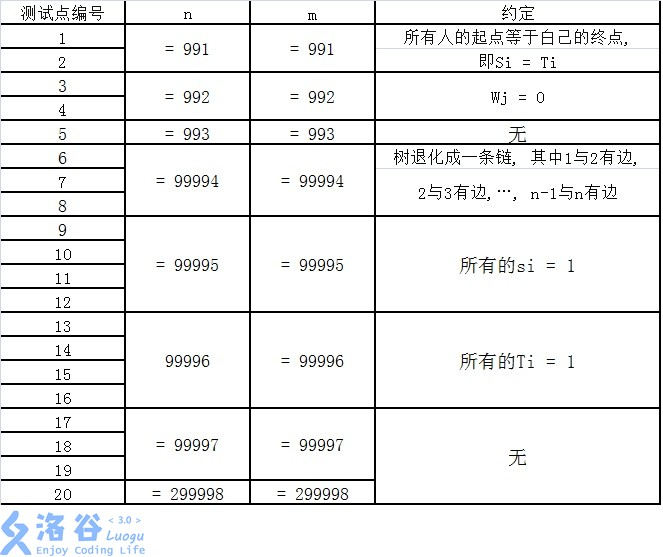# P1600 天天爱跑步

• 2.7K通过
• 18.1K提交
• 题目提供者 CCF_NOI
• 评测方式 云端评测
• 标签 Link-Cut Tree,LCT 差分 最近公共祖先,LCA 树链剖分,树剖 线段树 NOIp提高组 2016 高性能
• 难度 省选/NOI-
• 时空限制 2000ms / 512MB
• 提示：收藏到任务计划后，可在首页查看。

## 题目描述

小c同学认为跑步非常有趣,于是决定制作一款叫做《天天爱跑步》的游戏。《天天爱跑步》是一个养成类游戏,需要玩家每天按时上线,完成打卡任务。

这个游戏的地图可以看作一一棵包含 $n$个结点和 $n-1$条边的树, 每条边连接两个结点,且任意两个结点存在一条路径互相可达。树上结点编号为从$1$到$n$的连续正整数。

现在有$m$个玩家,第$i$个玩家的起点为 $S_i$,终点为 $T_i$ 。每天打卡任务开始时,所有玩家在第$0$秒同时从自己的起点出发, 以每秒跑一条边的速度, 不间断地沿着最短路径向着自己的终点跑去, 跑到终点后该玩家就算完成了打卡任务。 (由于地图是一棵树, 所以每个人的路径是唯一的)

小c想知道游戏的活跃度, 所以在每个结点上都放置了一个观察员。 在结点$j$的观察员会选择在第$W_j$秒观察玩家, 一个玩家能被这个观察员观察到当且仅当该玩家在第$W_j$秒也理到达了结点 $j$ 。 小C想知道每个观察员会观察到多少人?

注意: 我们认为一个玩家到达自己的终点后该玩家就会结束游戏, 他不能等待一 段时间后再被观察员观察到。 即对于把结点$j$作为终点的玩家: 若他在第$W_j$秒前到达终点,则在结点$j$的观察员不能观察到该玩家;若他正好在第$W_j$秒到达终点,则在结点$j$的观察员可以观察到这个玩家。

## 输入输出格式

输入格式：

第一行有两个整数$n$和$m$ 。其中$n$代表树的结点数量, 同时也是观察员的数量, $m$代表玩家的数量。

接下来 $n- 1$行每行两个整数$u$和 $v$,表示结点 $u$到结点 $v$有一条边。

接下来一行 $n$个整数,其中第$j$个整数为$W_j$ , 表示结点$j$出现观察员的时间。

接下来 $m$行,每行两个整数$S_i$,和$T_i$,表示一个玩家的起点和终点。

对于所有的数据,保证$1\leq S_i,T_i\leq n, 0\leq W_j\leq n$ 。

输出格式：

输出1行 $n$个整数,第$j$个整数表示结点$j$的观察员可以观察到多少人。

## 输入输出样例

输入样例#1： 复制
6 3
2 3
1 2
1 4
4 5
4 6
0 2 5 1 2 3
1 5
1 3
2 6 
输出样例#1： 复制
2 0 0 1 1 1
输入样例#2： 复制
5 3
1 2
2 3
2 4
1 5
0 1 0 3 0
3 1
1 4
5 5 
输出样例#2： 复制
1 2 1 0 1

## 说明

【样例1说明】

对于$1$号点，$W_i=0$，故只有起点为1号点的玩家才会被观察到，所以玩家$1$和玩家$2$被观察到，共有$2$人被观察到。

对于$2$号点，没有玩家在第$2$秒时在此结点，共$0$人被观察到。

对于$3$号点，没有玩家在第$5$秒时在此结点，共$0$人被观察到。

对于$4$号点，玩家$1$被观察到，共$1$人被观察到。

对于$5$号点，玩家$1$被观察到，共$1$人被观察到。

对于$6$号点，玩家$3$被观察到，共$1$人被观察到。

【子任务】

每个测试点的数据规模及特点如下表所示。 提示: 数据范围的个位上的数字可以帮助判断是哪一种数据类型。【提示】

如果你的程序需要用到较大的栈空问 (这通常意味着需要较深层数的递归), 请务必仔细阅读选手日录下的文本当rumung:/stact.p″, 以了解在最终评测时栈空问的限制与在当前工作环境下调整栈空问限制的方法。

在最终评测时，调用栈占用的空间大小不会有单独的限制，但在我们的工作环境中默认会有 $8 MB$ 的限制。 这可能会引起函数调用层数较多时， 程序发生栈溢出崩溃。

我们可以使用一些方法修改调用栈的大小限制。 例如， 在终端中输入下列命令 ulimit -s 1048576

此命令的意义是，将调用栈的大小限制修改为 $1 GB$。

例如，在选手目录建立如下 sample.cpp 或 sample.pas将上述源代码编译为可执行文件 sample 后，可以在终端中运行如下命令运行该程序

./sample

如果在没有使用命令“ ulimit -s 1048576”的情况下运行该程序， sample会因为栈溢出而崩溃； 如果使用了上述命令后运行该程序，该程序则不会崩溃。

特别地， 当你打开多个终端时， 它们并不会共享该命令， 你需要分别对它们运行该命令。

请注意， 调用栈占用的空间会计入总空间占用中， 和程序其他部分占用的内存共同受到内存限制。

提示
标程仅供做题后或实在无思路时参考。
请自觉、自律地使用该功能并请对自己的学习负责。
如果发现恶意抄袭标程，将按照I类违反进行处理。